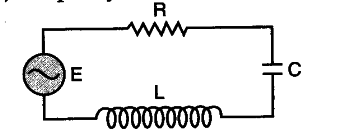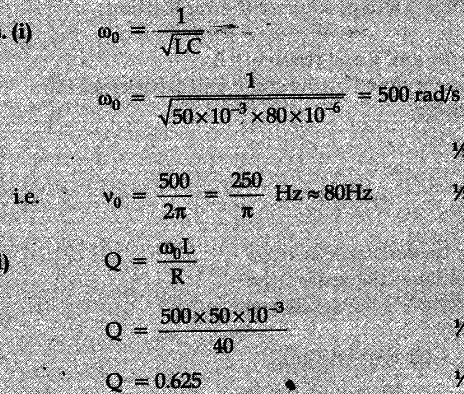# The figure shows a series LCR circuit connected to a variable frequency

The figure shows a series LCR circuit connected to a variable frequency of 200 V source with find
L = 50 mH, C = 80 muF and R = 40 ohm find.
(i) the source frequency which drives the circuit in
resonance;
(ii) the quality factor (Q) of the circuit